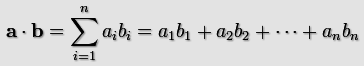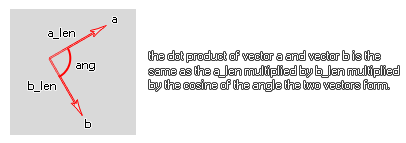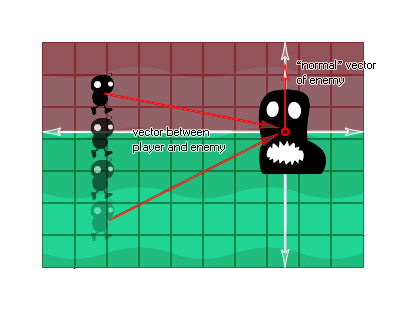## dot_product

### 语法：

dot_product(x1, y1, x2, y2)

x1 第一个向量的x坐标。
y1 第一个向量的y坐标。
x2 第二个向量的x坐标。
y2 第二个向量的y坐标。

### 描述a · b = (x1*x2)+(y1*y2);

a · b = (length of a) * (length of b) * cos(angle)• 如果 a 和 b 是垂直的 (在90度之间), 点积的结果将是零, 因为 cos (ang) 为零。
• 如果 a 和 b 之间的角度小于 90度, 点乘积将为正 (大于零), 因为 cos (ang) 将为正。
• 如果 a 和 b 之间的角度大于 90度, 点乘积将为负 (小于零), 因为 cos (ang) 将为负。### 例如：

var x1, y1, x2, y2;
x1 = lengthdir_x(1, image_angle);
y1 = lengthdir_y(1, image_angle);
x2 = o_Player.x - x;
y2 = o_Player.y - y;
if dot_product(x1, y1, x2, y2) > 0 seen=true else seen=false;